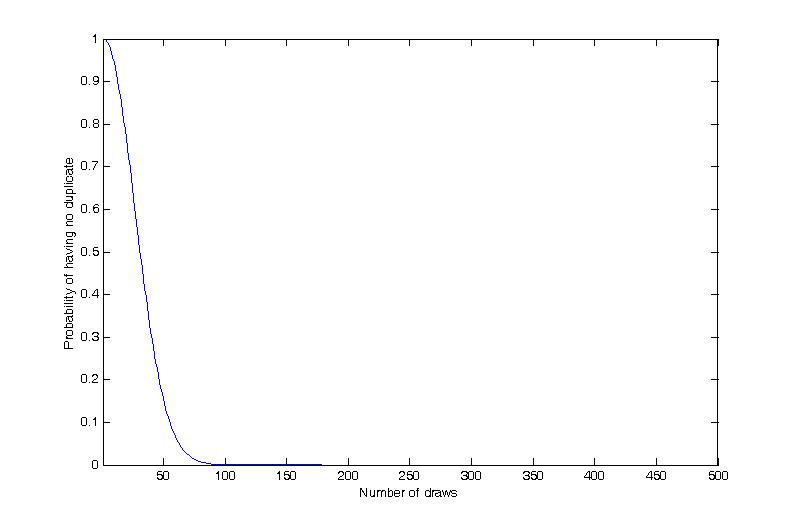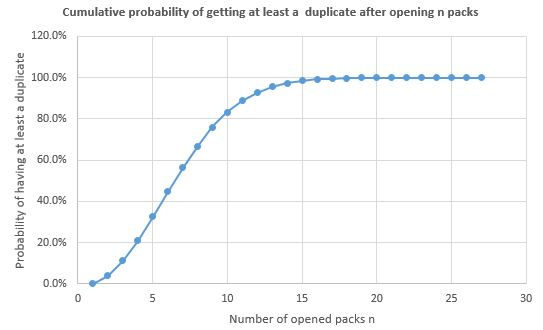# Monte-Carlo Method on Panini stickers distribution

Good afternoon everyone,

Today I want to write a funny post which combines two of the most important topics of June 2014: the CFA Level III curriculum and the FIFA World Cup. I know, that a long shot.

I assume that pretty much every reader of this blog had the opportunity to collect Panini stickers before major sporting events. The idea is quite simple. You have an album which you need to fill with stickers that you buy by packs. For the 2014 FIFA World Cup in Brazil, each pack contains 5 stickers and the album has roughly 650 stickers.When I was a child, I used to buy or to be offered I pack every day and filling the album took weeks. Today, I start off by buying a whole box of 100 packs, which makes it 500 stickers at a time. The thing is, as you open the packs, you would expect to have duplicate stickers that you will exchange with friends for stickers that you don’t already own. This year though, several of my friends and myself bought boxes and got absolutely no duplicate, which mean that we all had an album filled with 500 stickers straightaway.  It then looked clear to me that Panini did this on purpose, but I wanted to make sure using probability thoery.

# Question

What is the probability that, after 500 stickers, I still have no duplicate sticker?

Alternatively, you could ask: did Panini make sure that you have no duplicate if you buy a whole box of 500 stickers?

# Basic Assumption

For this experiment, I assume that getting the event of getting any sticker is equiprobable. This maximizes the chance of having no duplicate after several draws. Formally, I define a random variable $X$ which can take any value between 1 and $m$ (the number of existing stickers) and which represents the event of drawing the sticker’s number. Then we have that the probability of drawing sticker number $i$ is:

$$\mathbb{P}(X=i)=\frac{1}{m}$$

In our case, $m=650$ and the probability of getting any sticker is roughly 0.15%.

# Single draws

To begin, I remove the concept of packs and assume that I get every sticker randomly with the probability mentioned above. In this setup, it is quite easy to compute the probability of having no duplicate after $n$ draws:

After 1 draw, the probability of having a duplicate is 100%; of course, because you have no stickers at all.

After 2 draws, the probability of having no duplicate is:

$$\frac{650-1}{650}=\frac{649}{650}=99.85\%$$

The idea is simple: on the numerator is the number of cards that we don’t already have and on the denominator is the number of possible cards. When then multiply this probability by the probability of having no duplicate so far (which was 100%, i.e. 1, in this case).

After 3 draws we have:

$$99.85 \% \cdot \frac{650-2}{650}=99.54\%$$

We can generalize this by saying that for $m$ existing different stickers, the probability of having no duplicate after $n$ draws is:

$$\prod_{i=1}^n \frac{m – (i-1)}{m}$$

That’s rather easy to compute using any programming language such as Matlab or C#.

Coming back to our world cup 2014 example, we can draw the following chart to see how the probability of having no duplicate evolves along with the number of single draws performed:Probability of having no duplicate after n draws

As you can see, in this setup the probability of having no duplicate is almost 0% after 100 draws. So, there is little chance that this occurred if Panini did not make something special about it.

# Draws from packs

On argument that could be made is that you should not have any duplicate within a single pack. In this case, you are sure to have 5 distinct cards  every time you open a pack, and it should slightly boost your probability to have no duplicates after $n$ draws. This can be shown using a simple example: assume you draw 5 single cards, the probability of having no duplicate using the formula above gives 98.47%. If you get cards by pack, drawing 5 cards corresponds to drawing 1 pack, which by assumption guarantees having no duplicates (probability of 100%).

So, how do we compute the probability of having no duplicate after opening $n$ packs? Well I guess it is possible to formulate it mathematically, and frankly when I thought about this I was not really in the mood of digging into all these permutations. So, I decided to go with another approach: a monte-carlo simulation. How does that work? The idea is quite simple: I am going to create a computer program that simulates the process of opening packs of stickers. Here is how the program will work:

## Creating a pack

I assume the pack contains $p$ stickers, with an equal probability of drawing any sticker.

• Start with an empty pack
• As long as I don’t have $p$ stickers in the pack
• Draw a sticker randomly
• If the sticker is not already in the pack
• Add the sticker to the pack
• Return the full pack

## Running a simulation

Now that I have a procedure to create a pack, I will define what I mean by running a simulation. The idea is to simulate the action of opening packs randomly generated on the fly until I get a duplicate in the stickers I got:

• Start with an empty album
• Until I have a duplicate a duplicate sticker
• Generate a random pack
• Open the pack and put the stickers in the album
• Return the number of opened packs

So, what I’m doing here is to simulate a random variable $Y$ which is the number of opened pack before getting a duplicate.

Back to our Panini example, we really simulate opening packs as we do from the box of 100 packs. What we would like to do is to estimate the probability distribution of this variable $Y$ in order to be able to discuss whether it is statistically possible that we got no duplicate after 100 packs. This is where Monte-Carlo simulation comes into action.

## Monte-Carlo simulation

The principle of Monte-Carlo simulation is to simulate the outcome of a random variable $k$ times, where $k$ is very large. We divide the number of each outcome we got  by the total number of simulations, $k$. This gives us an estimation of the probability of each outcome.

This is exactly what I did for the Panini example, and I got the following results:Probability of having first duplicate after opening n packs.

In the graph above, you see the probability of having the first duplicate after opening $n$ packs, i.e. $\mathbb{P}(Y=n)$. To be able to make a final assessment, we would like to see the estimated cumulative distribution of $Y$ which corresponds to the probability of having at list a duplicate after opening $n$ packs:Cumulative Probability of having at least one duplicate after opening n pack.

As you can see, after 15 packs, there is a probability of having no duplicate of almost zero. That is, a probability of about 0 of having no duplicate after $5 \cdot 15 = 75$ stickers. This means that there is almost no chance that my friends and I had no duplicate after opening a whole box of 100 packs.

So, there is not much difference with the simple model, but that’s not really the point. The idea is that it was hard to get a closed form of the probability of having at least duplicate after $n$ packs, but that by using the Monte-Carlo simulation, we managed to estimate this. This is sometimes also used for simulating scenarios under some model assumptions!

I hope you enjoyed this funny example!

Cheers,

Jeremie